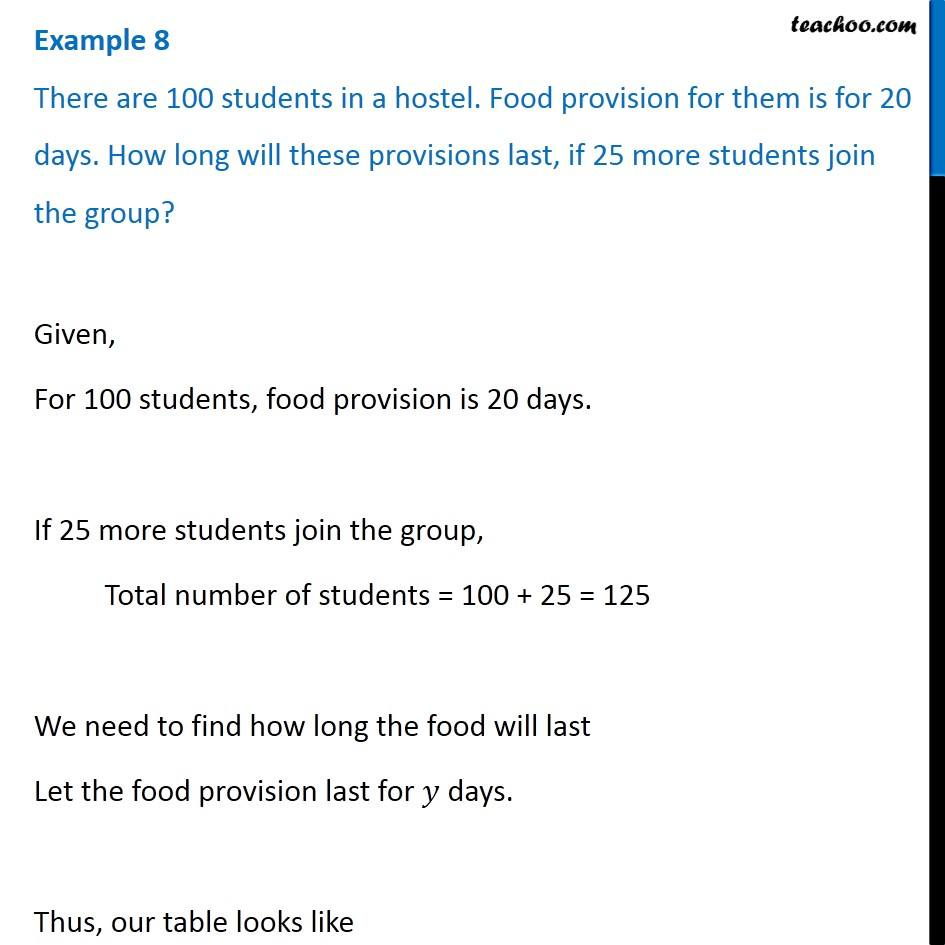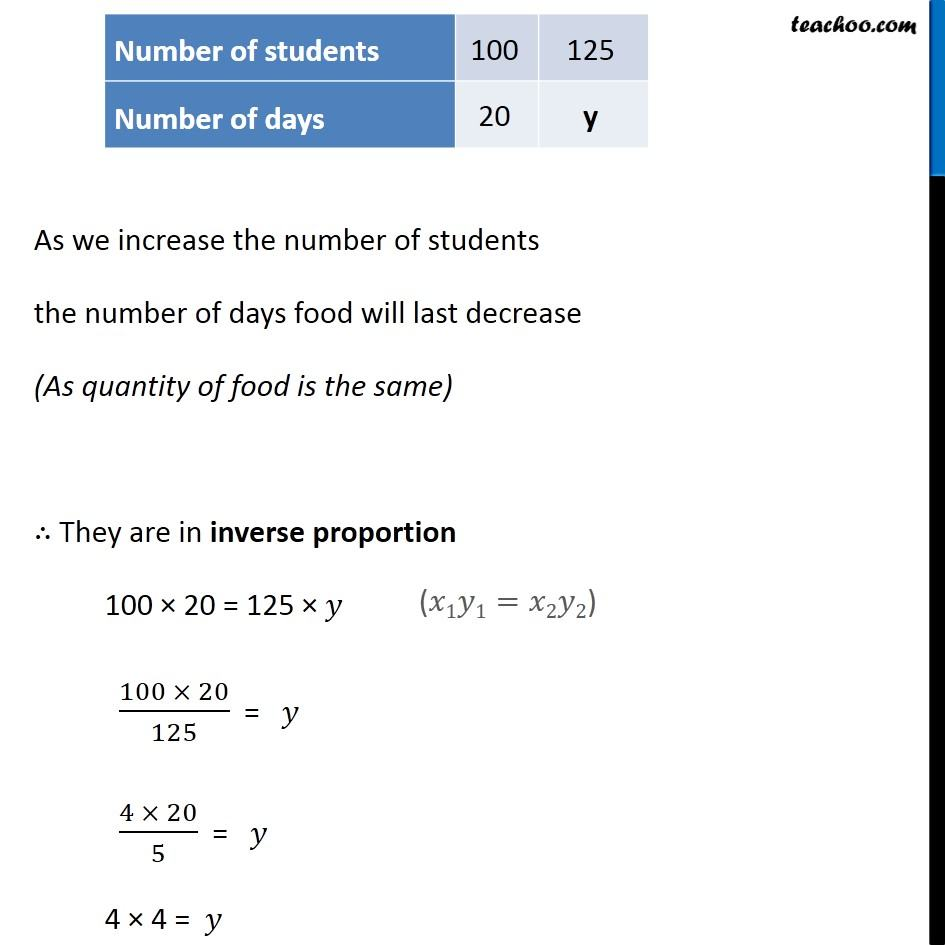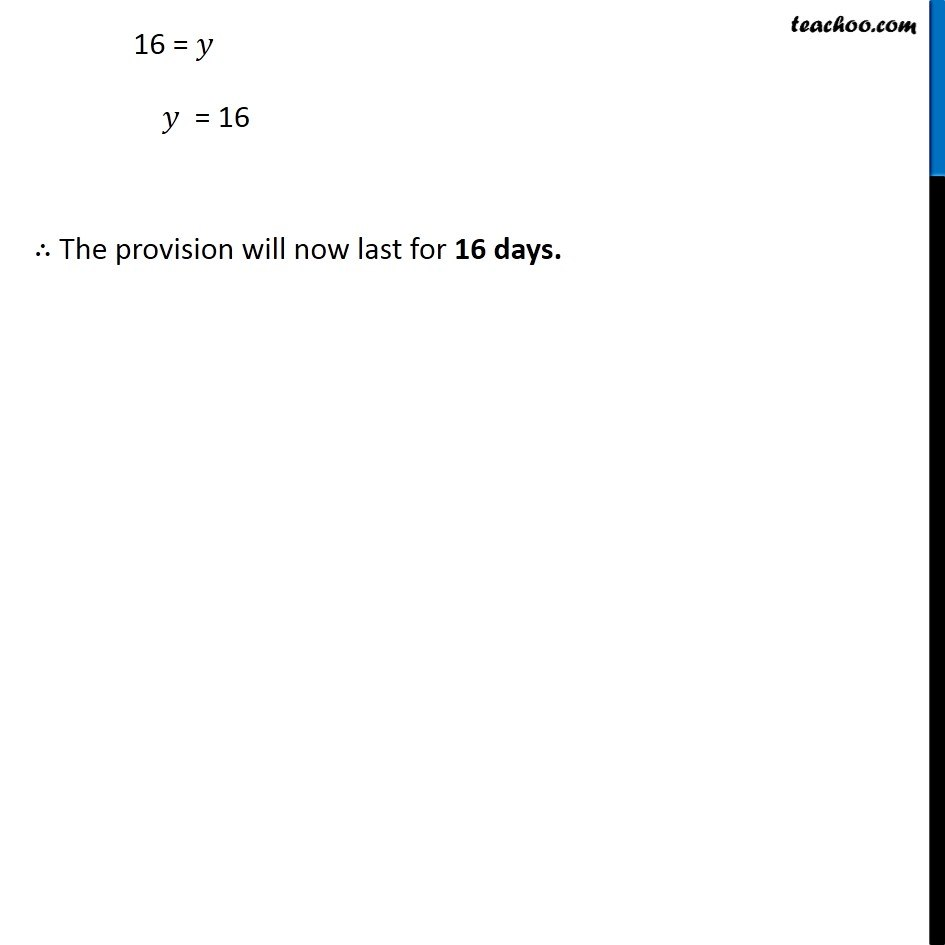Inverse Proportion

Chapter 13 Class 8 Direct and Inverse Proportions
Concept wise### Transcript

Example 8 There are 100 students in a hostel. Food provision for them is for 20 days. How long will these provisions last, if 25 more students join the group? Given, For 100 students, food provision is 20 days. If 25 more students join the group, Total number of students = 100 + 25 = 125 We need to find how long the food will last Let the food provision last for 𝑦 days. Thus, our table looks like As we increase the number of students the number of days food will last decrease (As quantity of food is the same) ∴ They are in inverse proportion 100 × 20 = 125 × 𝑦 (100 × 20)/125 = 𝑦 (4 × 20)/5 = 𝑦 4 × 4 = 𝑦 16 = 𝑦 𝑦 = 16 ∴ The provision will now last for 16 days.